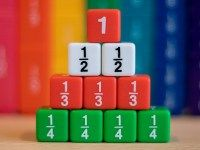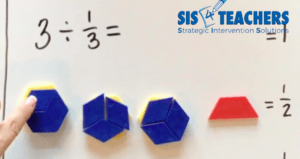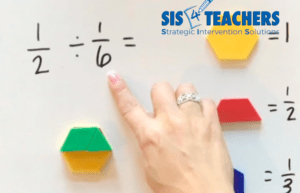Select Page

# Working with Fractions: Dividing Fractions

Mar 12, 2020

For most of us dividing fraction is one of those things where we got the right answer in math, but we don’t really remember why.Most of us memorized a procedure with a concept we don’t understand: “don’t ask why, just invert and multiply.” Maybe it’s time to ask why!!

When we look at dividing fractions with our students today, we can start with a lot of questions that challenge that memorized procedure: Is it possible to actually divide by fractions or can you only multiply by fractions? And why do we flip or invert fractions and then multiply??

I was in a classroom not too long ago, modeling the concepts we’re talking about today by using the pattern blocks to help students explore their knowledge, and I asked the students why do we invert and multiply  (or “keep, change, flip” in some classrooms)?

A student raised his hand and said, So you can get more of an accurate answer. I responded by asking Is it going to be inaccurate if you divide??

In today’s blog, we’re going to explore how we should have learned about dividing fractions before we learned a trick to get us the right answer.

## A Foundation of DivisionIt’s really important that students actually understand really what’s happening in division with whole numbers before they jump into division of fractions, or numbers less than one. As we look at this dividing fractions, we can start by asking students about a statement for division: If I had 35 ÷ 6, what does that mean? A lot of kids, just like we talked about in multiplication, might say you’re dividing, or they might even tell you the answer. But what that question is really asking is how many groups of 6 are in 35, or how many 6 are there in 35? This helps students revisit their prior knowledge of division, but also helps them extend that knowledge as we start dividing whole numbers by a fraction.

Another thing to think about with division is the relationship with multiplication. Like we have fact family with whole numbers, we want to help kids see how, when you flip multiplication and division, there’s an inverse relationship there. If I have 12 ÷ 4, it goes in 3 times, just like if I have 3 × 4, I know that it equals 12. Understanding this relationship between multiplication and division with whole numbers will help students make further connections when we are looking at fractions.

## Whole Number Divided by a Fraction

A students begin to process division of fractions, we can start with asking them to think about the idea of division as applied to fractions: When we divide by a whole number, we end up with a smaller number. But when we divide by a fraction, it actually increases the size of the answer – why is that? Why is it that, when we divide fractions we can end up with a whole number if it goes in an equal amount of groups?

In this video, I model dividing a whole number by a fraction, and I use the pattern blocks to give students a visual manipulative to help solidify the concept for them. We’ll be using the hexagon as a whole, the trapezoid as ½, our rhombus as ⅓, and then our triangle as ⅙.So, let’s take 3 ÷ ⅓ – what is that asking? It’s asking how many ⅓ are there in 3? I’ll start by building 3 with 3 hexagons so kids can see it represented, and then ask how many ⅓ do you see in there?  Students can lay the rhombus on top of those 3 hexagons to see how many actually fit in 3. If I covered each whole with 3 rhombuses, I would know for every 1 whole, there are 3 rhombuses, so 3 ÷ ⅓ = 9.

Let’s relate this problem to multiplication. If I were to change the problem around and multiply, would I end up with the same answer? 9 × ⅓  is asking what is 9 groups of ? The answer is 3, as we can see if we build it with the pattern blocks. This is the same kind of fact family relationship that we might understand with whole numbers with multiplication or division.

## Dividing a Fraction by a Fraction

When we start thinking about dividing a fraction by a fraction, it’s important to keep the same language that you’ve been using with whole numbers in division, or even with a whole number divided by a fraction.

Some fractions, when you divide them, go in nice and evenly. I definitely suggest starting with this kind of friendly unit fractions at first to help students understand dividing a fraction by a fraction. Fractions that divide evenly without a remainder or something that is less than one will help students develop a more conceptual understanding at first.Let’s start with ½ ÷ ⅙ . What that’s asking is how many ⅙ are there in ½ ? When using the pattern blocks, I like to put the hexagon down so kids always have that reference for the whole that we’re working with. I’ll start with ½ piece and ask how many ⅙  will fit into ½?

We can see that, if we took the ⅙ pieces and we tried to fit them inside of the ½, we know that three of those would fit. So we could say that three ⅙ pieces will fit evenly on the ½, which will give us a whole number (3) as our answer.

Other fraction examples might fit more than one. So let’s look at ⅚, and ask how many ⅓ can fit in ⅚? Or  ⅚ ÷ ⅓ . First, we want to build the ⅚, so I put ⅚, 5 triangle pieces, on top of the hexagon. We know that if we added one more ⅙, it would be nice and even. But in this case, we’re working with  ⅚. Then, I take my rhombus, which is equal to ⅓, to try and figure out how many times it will fit.

I see that ⅓ is equal to 2/6, and if I have another 2/6, I will have another ⅓. By using the rhombus, I can see that ⅓ goes into ⅚ two times, but with ⅙ leftover. This is where students often get confused in Division. They see that there’s another ⅙ left and think that’s the remainder. But we have to remember that we’re really dividing by ⅓, so we have to look at any fractional parts in relation to that amount.

If there’s ⅙ left, we still want to know how many ⅓ will fit into it. A whole ⅓ doesn’t fit, but a half of ⅓ does.  So, ⅚ ÷ ⅓ = 2 ½.

## Less than a One

The other thing we show in the video is a situation when something might be less than one.

If we were to ask how many ½ are there in ⅓ , which we’ve written out as ⅓ ÷ ½, we know that we might not be able to figure that out completely. In the video, you can see it’s especially challenging to look at this in 6th grade when they see that ½ doesn’t even go into ⅓ one whole time. The answer is less than one time.

In the video we compare the trapezoid with the rhombus and show kids that it doesn’t even go in one time. But if I took that ½ and divided it into three sections, how much of it does fit? This is a little bit more higher level thinking, and it could throw even some of the teachers that we work with for a loop. It’s very hard to visualize this because we didn’t learn this way as children.

Visually, we can stack that ⅓ on top of ½ so kids could see the ½ doesn’t fit completely. However,  if we divide the ⅓ up into three equal sections, ⅔ of that ½ does actually fit. As confusing as it sounds, the video shows how kids could visualize or see that based on the pieces that they’re using!

## Invert and Multiply

The next thing you really want students to understand is why we flip or invert the fractions and multiply? Can I create a multiplication sentence that will be true for the fact family? Does that work only with a whole number and a fraction or can you do it with the fraction and a fraction?

That is one of those open ended things we want your kids to explore instead of you answering the question for them. I would rather your kids see why it works when you invert and multiply and then see it would still work the same way with division and using the language appropriately. In offering inquiry-based questions, you don’t necessarily have to be the giver of all the information. Getting kids to make that connection and deepen their understanding is what we’re looking for in the 21st century math instruction.

## Why before HowUsing our Working with Fractions series videos – both multiplication and division so far! – your students will really start to understand the why before the how when it comes to fractions. If we can get kids to understand what they’re really doing and have a deep conceptual understanding for it, they’re better able to explain.

Today’s questions on 21st century tests no longer just as students to compute the answer. It often makes students draw a picture to show a problem like 4 ÷ ½, and show their understanding of what they’re doing, not just that they can regurgitate the information.

These YouTube videos are really great in a flipped classroom style setting. Whether you’re doing it in the launch of your lesson, or you’re actually having the students listen to this at home, or maybe even in a guided group with other peers, they could listen to this video before coming to see you. The 21st century mind needs that technological dip, even as much as I try to fight it, Marzano’s research really supports this idea that, if we could get kids to see something in video and interact with it hands on, and then apply it, it makes all the difference.

Next week we’ll be talking about the addition of fractions using a conceptual way as well to show how we can reduce fractions using one of our favorite friends, DC from Mathville. Check it out!